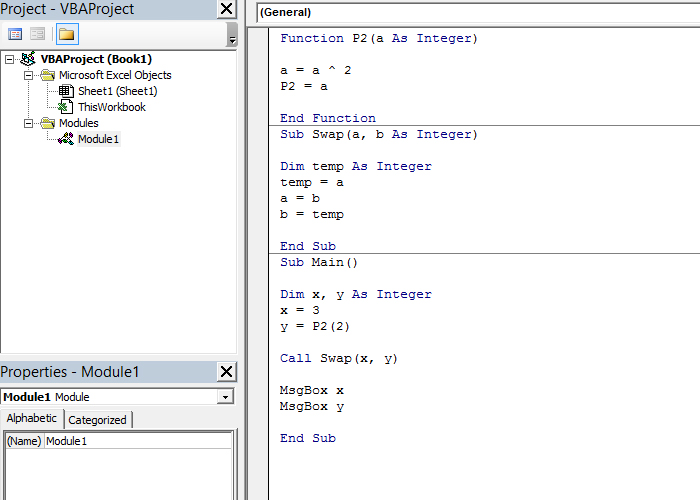# Calling a subroutine

During program development it’s important to notice which of its parts can be reused and, based on that fact, should create subroutines that will be called within the main program. Subroutines can be functions and procedures. We use the functions by specifying the variable name, followed by equality sign, and then the name of the function with the arguments. We invoke the procedures using the Call command, after which we specify the procedure name with the arguments.

I have created one simple application consisting of the main program (Main) that calls the function (P2) and the procedure (Swap). The function P2 calculates square of given argument variable, while the Swap function changes the places of the two given variables. In the main program, we first need to assign values to two variables, where the second is square of number two. Then we invoke the Swap procedure in order to replace their places, and we see the result after displaying their values.

Function P2 (a As Integer)

a = a ^ 2
P2 = a

End Function

Sub Swap (a, b As Integer)

Dim temp As Integer
temp = a
a = b
b = temp

End Sub

Sub Main ()

Dim x, y As Integer
x = 3
y = P2(2)
Call Swap(x, y)
MsgBox x
MsgBox y

End Sub